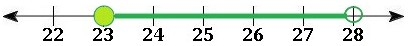Systems for Expressing Sets

Systems for Expressing Sets

A set is an assortment of distinctive components that do not repeat. Sets can be defined in different forms. Here are four common methods of interpreting them.

Set-Builder Notation

Set-builder notation is a mathematical coding system that shows the properties that the components of a set need to satisfy. A simple example is written as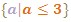which is expressed as the set ("{  }") of all a ("a"),such that (" | ") a is less than or equal to three ("a ≤ 3"), which can also be expressed as any value less than or equal to 3.

Example

Describe the following set: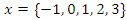In set-builder notation, this set is written as: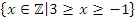This set is described as all integer numbers that are less than or equal to three and equal to or greater than negative one, or all integer numbers between -1 and 3 inclusive.  The bold "Z" in the expression indicates that the x-values in the set are limited to the integers.

Here is another example: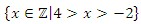This set is described as all integer numbers that are between four and negative two, non-inclusive.

Roster Notation

A roster is a list of the elements in a set. They are surrounded by braces and separated by commas.

Example

Write in roster notation all the integer numbers that are greater than 0 and less than 10, non-inclusive: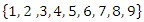Write in roster notation all integer numbers that are less than two, inclusive.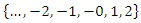Interval and Graphical Notation

An interval is a connected subset of numbers, an alternative to expressing the values as an inequality. When using interval notation we use two types of symbols:

" which means non-inclusive or open.

" [ " which means inclusive or closed.

Here’s how you would describe a set with interval notation: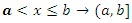This set represents all numbers between a and b, including b but not including a.

You can show an interval on a graph as well.  Here are six types of interval notation and how they generally look on a number line: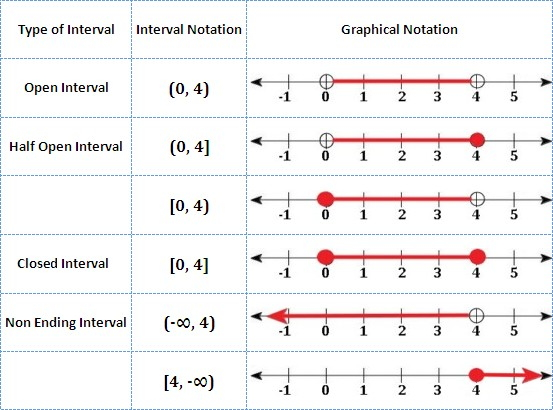Notice how interval notation and graphical notation always include all numbers in their sets, not just the integers.  For example, 3.2 is an element of the set [2, 5], but not the set {2, 3, 4, 5}.

Example

Write this notation for all integers between 23, inclusive, and 28, non-inclusive.

Roster Notation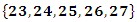Set-Builder Notation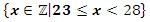Write this notation for all numbers between 23, inclusive, and 28, non-inclusive.

Interval Notation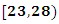Graphical Notation